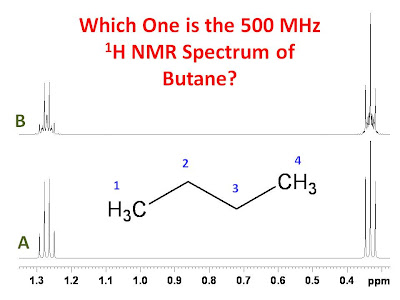## University of Ottawa NMR Facility Web Site

Please feel free to make suggestions for future posts by emailing Glenn Facey.

## Tuesday, August 4, 2009

### The 500 MHz 1H NMR Spectrum of Butane

n-Butane is a very simple molecule. Should it not then give a very simple 1H NMR spectrum? The figure below shows two calculated 500 MHz proton spectra for n-butane. Which spectrum most closely represents the true spectrum of n-butane?The answer is the very complicated spectrum B. The spectra were calculated with the following parameters:

Spectrum B
Spectrometer frequency = 500 MHz
δ1 = δ4 = 0.333 ppm
δ2 = δ3 = 1.271 ppm
3J12 = 3J34 = 7.11 Hz
4J13 = 4J24 = -0.07 Hz
3J23 = 6.77 Hz
LB = 0.5 Hz

Spectrum A
Spectrometer frequency = 500 MHz
δ1 = δ4 = 0.333 ppm
δ2 = δ3 = 1.271 ppm
3J12 = 3J34 = 7.11 Hz
4J13 = 4J24 = -0.07 Hz
3J23 = 0 Hz
LB = 0.5 Hz

The only difference between the simulations is that in spectrum B a coupling of 6.77 Hz was assumed between the two methylene groups whereas in spectrum A the same coupling was taken to be zero. The reason spectrum spectrum B is so complicated is that despite the fact that both the methyl groups and both the methylene groups are chemically equivalent, they are not magnetically equivalent. This is true for both spectrum A and spectrum B however, in spectrum A the second order effects are small based on the parameters used in the simulation.

Thank you to Adrian Dingle for inspiring me to create this post.